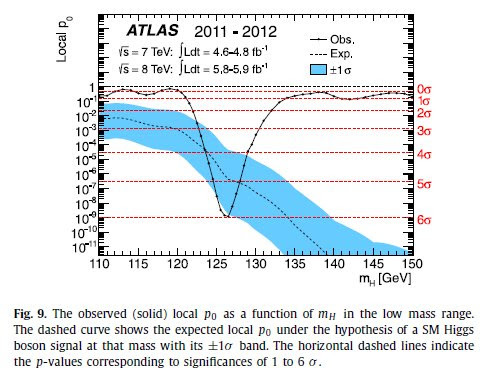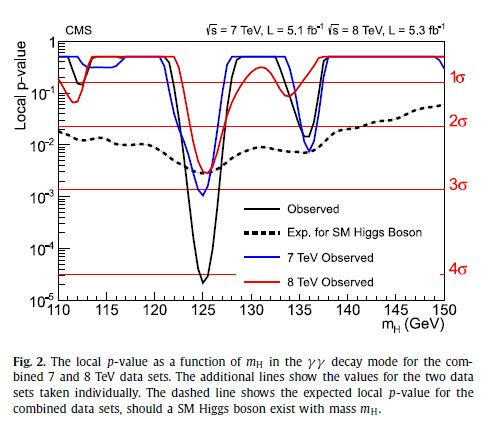### Habemus papers (about the new boson)

Finally Physics Letters B published the two papers by ATLAS and CMS about the discovery of the new boson at LHC (via tanzmax):A search for the Standard Model Higgs boson in proton–proton collisions with the ATLAS detector at the LHC is presented. The datasets used correspond to integrated luminosities of approximately $4.8 \, fb^{−1}$ collected at $\sqrt{s} = 7$ TeV in 2011 and $5.8 \, fb^{−1}$ at $\sqrt{s} = 8$ TeV in 2012. Individual searches in the channels $H \rightarrow ZZ^{(*)} \rightarrow 4l$, $H \rightarrow \gamma \gamma$ and $H \rightarrow WW^{(*)} \rightarrow e \nu \mu \nu$ in the 8 TeV data are combined with previously published results of searches for $H \rightarrow ZZ^{(*)}$, $WW^{(*)}$, $b \bar{b}$ and $\tau^+ \tau^-$ in the 7 TeV data and results from improved analyses of the $H \rightarrow ZZ^{(*)} \rightarrow 4l$ and $H \rightarrow \gamma \gamma$ channels in the 7 TeV data. Clear evidence for the production of a neutral boson with a measured mass of 126.0 ± 0.4 (stat) ± 0.4 (sys) GeV is presented. This observation, which has a significance of 5.9 standard deviations, corresponding to a background fluctuation probability of 1.7 × 10−9, is compatible with the production and decay of the Standard Model Higgs boson.
Aad, G., Abajyan, T., Abbott, B., Abdallah, J., Abdel Khalek, S., Abdelalim, A.A., Abdinov, O., Aben, R., Abi, B., Abolins, M. & (2012). Observation of a new particle in the search for the Standard Model Higgs boson with the ATLAS detector at the LHC, Physics Letters B, 716 (1) 29. DOI: 10.1016/j.physletb.2012.08.020Results are presented from searches for the standard model Higgs boson in proton–proton collisions at $\sqrt{s} =$ 7 and 8 TeV in the Compact Muon Solenoid experiment at the LHC, using data samples corresponding to integrated luminosities of up to $5.1 fb^{−1}$ at 7 TeV and $5.3 fb^{−1}$ at 8 TeV. The search is performed in five decay modes: $\gamma \gamma$, $ZZ$, $W^+ W^−$, $\tau^+ \tau^-$, and $b \bar{b}$. An excess of events is observed above the expected background, with a local significance of 5.0 standard deviations, at a mass near 125 GeV, signalling the production of a new particle. The expected significance for a standard model Higgs boson of that mass is 5.8 standard deviations. The excess is most significant in the two decay modes with the best mass resolution, $\gamma \gamma$ and $ZZ$; a fit to these signals gives a mass of 125.3 ± 0.4 (stat.) ± 0.5 (syst.) GeV. The decay to two photons indicates that the new particle is a boson with spin different from one.
Chatrchyan, S., Khachatryan, V., Sirunyan, A.M., Tumasyan, A., Adam, W., Aguilo, E., Bergauer, T., Dragicevic, M., Erö, J., Fabjan, C. & (2012). Observation of a new boson at a mass of 125 GeV with the CMS experiment at the LHC, Physics Letters B, 716 (1) 61. DOI: 10.1016/j.physletb.2012.08.021

To the previous papers, I add also the following (via spimpompam), that it could be interesting to read:
Following recent ATLAS and CMS publications we interpret the results of their Higgs searches in terms of Standard Model operators. For a Higgs mass of 125 GeV we determine several Higgs couplings from 2011 data and extrapolate the results towards different scenarios of LHC running. Even though our analysis is limited by low statistics we already derive meaningful constraints on modified Higgs sectors.
Klute, M., Lafaye, R., Plehn, T., Rauch, M. & Zerwas, D. (2012). Measuring Higgs Couplings from LHC Data, Physical Review Letters, 109 (10) DOI: 10.1103/PhysRevLett.109.101801 (arXiv)